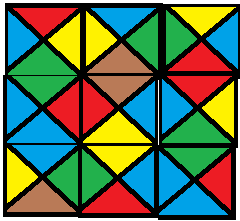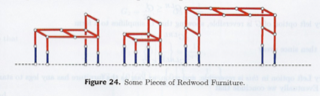## Square Tiling

The reference in G&J is to an “unpublished result” (by Garey and Johnson themselves, with Papadimitriou).  I think the solution I found is not the one they are referring to.

The problem: Square Tiling.  This is problem GP13 in the appendix.

The description: Given a set C of colors, and a set T of tiles, where each of the 4 sides of the tile are colors from C (listed as a 4-tuple of (top, right, bottom, left) colors), and an integer N.  Can we tile an NxN square of tiles using tiles from C?  We can use a tile more than once, the tiles can’t be rotated, and adjacent edges need to match colors.

Example: Here are some example tiles I drew:We can tile these into a 3×3 grid like this:Reduction: As I said above, the reference in G&J is to an “unpublished result” by Garey, Johnson, and Papadimitriou.  I did manage to find a “generic reduction” using Turing Machines in the Lewis and Papadimitriou Theory book.

The reduction is from the “N2” language in the book, which (I think) is “Can a Turing Machine M halt in t steps or less with its head on the tape in configration uσv, where u and v are strings in Σ*, and σ is the location of the head (and a symbol in the alphabet)?

The idea is that we’ll build a set of tiles whose colors are based on the number of steps the computation has done so far.  The colors are actually tuples.  So, we have several kinds of tiles:

• For each a in Σ*, and each k from 1 to t, a tile with color (a, k+1) on the top, and (a,k) on the bottom.  This simulates a move that stays in the same state and writes the same symbol.
• For each pair a,b in Σ*, and each state p,q in M (p can include the halt state, q can’t), a tile with the color (p,b,k+1) on the top and (q,a,k) on the bottom.  This simulates going from state p to state q and replacing an a with a b.
• We can transition from one of the above types of tiles to another using a sideways move.  (If the head moves left or right, we move to a tile to the left or right)
• There are special tiles for the start row and for when the machine halts.

We set our N (the size of the tiling grid we’re making) to t+2.  What we’ve built is a system that:

• Has to have the tiles corresponding to one of the start states on the bottom row
• Has in row i a tile corresponding to a configuration of the machine after i steps.  (A path of tiles from the bottom to row i show the computation needed to get to that state)
• Has at the top row a tile corresponding to a configuration after t+1 steps.  If there is a legal tiling, one of those tiles must contain the halt state.

..which they claim is the same as the N2 langauge.

The suggested reduction in the appendix is from Hamiltonian Path, and I spent some time thinking about how to make that work, but couldn’t do it.  Do you make tiles correspond to vertices? Do you make colors correspond to vertices?  How do you account for the fact that you can go in two dimensions?  How do you account for the fact that you don’t know the order in which you visit the tiles?  I think it might be similar to this way of thinking about configurations.

Difficulty: 9 because it’s such a non-standard way of doing things.  I bet the Hamiltonian Path reduction is a lot easier.

## Left-Right Hackenbush for Redwood Furniture

Here’s an interesting problem, but a hard one to explain.  Most of what I’m doing here comes from the very cool “Winning Ways for Your Mathematical Plays” book by Berlekamp, Conway, and Guy, which I hope at some point in the future to have the time to really dig deeply into.  But for now, I’ll just use it as a reference to this week’s problem.

The problem: Left-Right Hackenbush for Redwood Furniture.  This is problem GP12 in the appendix.

The description: Ok, here we go.  First, a Hackenbush problem consists of an undirected, connected graph.  The edges of the graph are marked as “Left” or “Right”(though the book has some very nice colored pictures, where the edges are labeled “Blue” and “Red”).  Some of the vertices are on the ground (In G&J’s definition, there is one ground vertex, but it’s equivalent to having several vertices that are all on the ground).

On Left’s turn, they remove a blue edge and then all edges that are not connected to the ground are removed.  On Right’s turn, they remove a red edge, and then all edges that are not connected to the ground are removed.  A player loses if there are no remaining edges of their color.

redwood furniture Hackenbush instance is one where:

• No red edges touch the ground
• Each blue edge (or “foot”) has one end on the ground and the other touches a unique red edge (the “leg”)

Here are some redwood furniture instances from the book:The “value” of a Hackenbush position is the number of “spare” moves (with optimal play) one player has after the other player loses.  A value of 0 means that whoever’s turn it is will lose (on an empty board).  The definition can be extended to fractional values.  For example, a value of 1/2 for (say) Left means that if we made two copies of the game, we would end up with a situation with a value of 1 for Left.

So, the question is, for some Redwood Furniture graph, and some K, is the value <= 2-K?

Reduction:

I’m just going to sketch the process here since it takes several pages of the book (and depends on results and ideas from earlier in the book).

They show:

• The value of any redwood furniture graph is 2-N for some N.  In the degenerate case, the graph with just one Left edge has a value of 1. (=20)
• On Left’s turn, they will always remove a foot (by definition, that’s all they have).  On Right’s turn, they should make a move that does not disconnect the graph, if possible.
• A “Bed” is a redwood furniture graph where every red edge that is not a leg connects to a leg.  It has value 2-(m+1), where m is the largest number of moves that do not disconnect the bed.
• The value of the game depends on how many extra moves Red has to get down to just a bed.
• To find m, you need to know the size of the largest redwood tree (a tree is a graph that will be disconnected by the removal of any edge) that contains all of the legs.
• The edges of the bed (the red edges that are not legs) form a bipartite graph.  So finding m is equivalent to the set covering problem, where the elements of the set are the vertices, and the edges are the sets.

Here’s how I think the Set Covering reduction works.  Given a set covering instance: a set S of elements, and a collection C of subsets of S, we’ll build a bipartite graph.  One set of the bipartite graph will correspond to the elements of S (and will be on the legs of the furniture graph).  The other set will correspond to the elements in C (and will be the vertices in the bed that are not in the legs).  An edge will go from each “C vertex” to each “S vertex” in its set.  Now, the cover is the set of vertices from the bed that cover all of the legs.

The book says you want the “smallest redwood tree which contains all of the legs”, which I think is the same thing (smallest number of extra vertices), but I’m not 100% confident since the Hackenbush game involves removing edges, and we’re choosing vertices in the cover.

I’m a little sad that the book does such a great job describing the game, and the value function, and then glosses over the reduction part (and uses misleading terms like “Minimum Spanning Tree of a Bipartite Graph”, which is a polynomial problem).  The actual reduction in G&J is to a private communication, so that’s not much help.

Difficulty: Boy, I don’t know, I think it depends on where you start from.  If my Set Cover reduction is the right one, and all you ask a student to do is that, it’s probably a 4.  If you’re going to make them prove all of the things I glossed over about the value number, then it probably goes up to at least an 8.